# CS 121 Digital Logic Design Chapter 3 GateLevel

• Slides: 61CS 121 Digital Logic Design Chapter 3 Gate-Level Minimization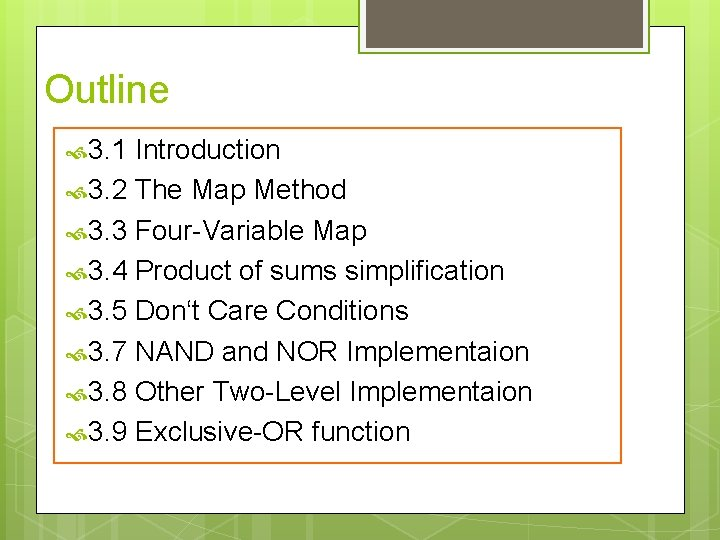Outline 3. 1 Introduction 3. 2 The Map Method 3. 3 Four-Variable Map 3. 4 Product of sums simplification 3. 5 Don‘t Care Conditions 3. 7 NAND and NOR Implementaion 3. 8 Other Two-Level Implementaion 3. 9 Exclusive-OR function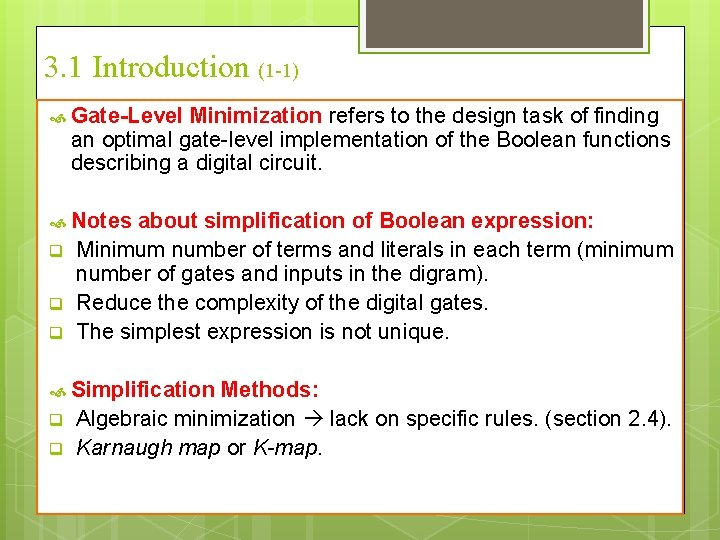3. 1 Introduction (1 -1) Gate-Level Minimization refers to the design task of finding an optimal gate-level implementation of the Boolean functions describing a digital circuit. Notes q q q about simplification of Boolean expression: Minimum number of terms and literals in each term (minimum number of gates and inputs in the digram). Reduce the complexity of the digital gates. The simplest expression is not unique. Simplification q q Methods: Algebraic minimization lack on specific rules. (section 2. 4). Karnaugh map or K-map.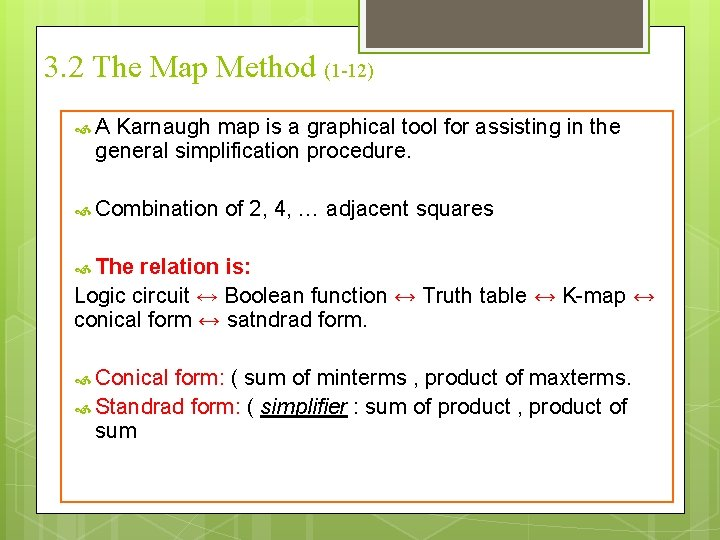3. 2 The Map Method (1 -12) A Karnaugh map is a graphical tool for assisting in the general simplification procedure. Combination of 2, 4, … adjacent squares The relation is: Logic circuit ↔ Boolean function ↔ Truth table ↔ K-map ↔ conical form ↔ satndrad form. Conical form: ( sum of minterms , product of maxterms. Standrad form: ( simplifier : sum of product , product of sum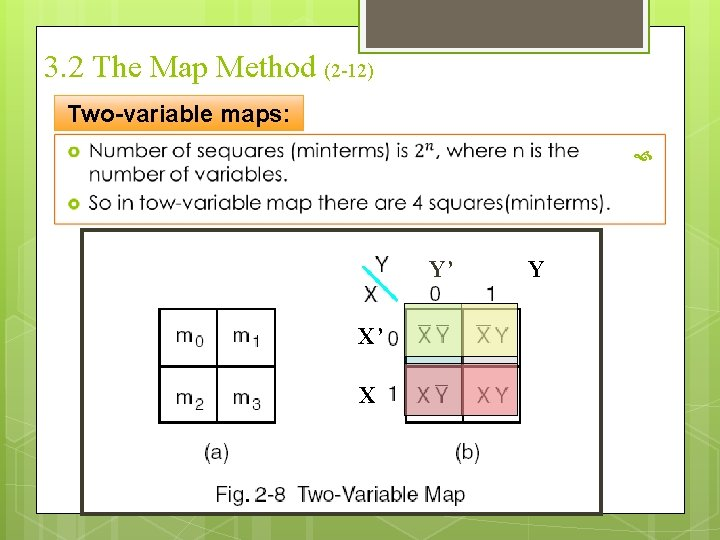3. 2 The Map Method (2 -12) Two-variable maps: Y’ X’ X Y3. 2 The Map Method (3 -12) Rules for K-map: o We can reduce functions by circling 1’s in the K-map o Each circle represents a minterm reduction o Following circling, we can deduce minimized and-or form. q Rules to consider o Every cell containing a 1 must be included at least once. o The largest possible “power of 2 rectangle” must be enclosed. o The 1’s must be enclosed in the smallest possible number of rectangles.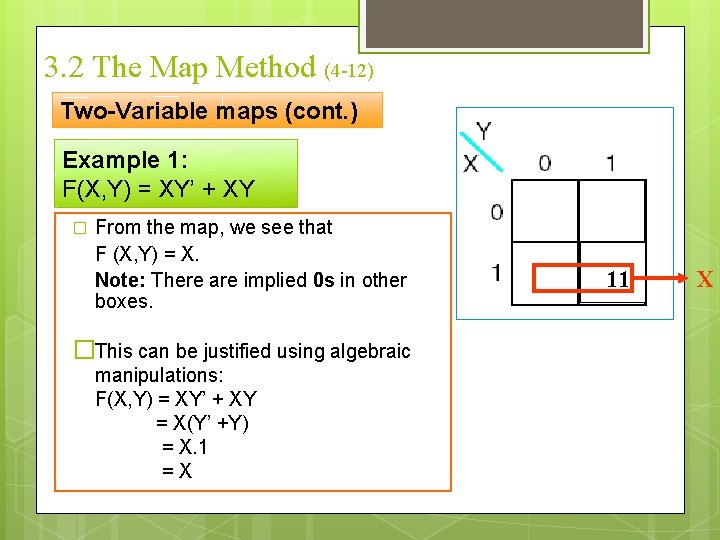3. 2 The Map Method (4 -12) Two-Variable maps (cont. ) Example 1: F(X, Y) = XY’ + XY � From the map, we see that F (X, Y) = X. Note: There are implied 0 s in other boxes. �This can be justified using algebraic manipulations: F(X, Y) = XY’ + XY = X(Y’ +Y) = X. 1 =X 11 X3. 2 The Map Method (5 -12) Two-Variable maps (cont. ) Example 2: Y G(x, y) = m 1 + m 2 + m 3 � G(x, y) = m 1 + m 2 + m 3 = X’Y + XY’ + XY � From the map, we can see that : G=X+Y 1 1 1 X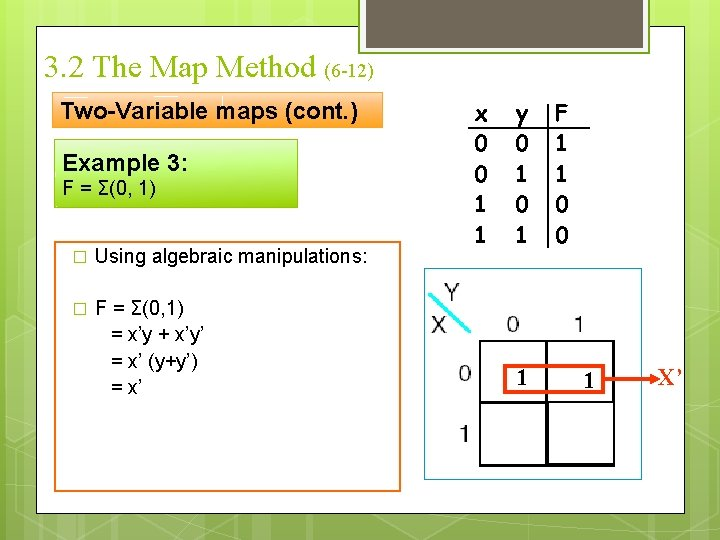3. 2 The Map Method (6 -12) Two-Variable maps (cont. ) Example 3: F = Σ(0, 1) � Using algebraic manipulations: � F = Σ(0, 1) = x’y + x’y’ = x’ (y+y’) = x’ x 0 0 1 1 y 0 1 1 F 1 1 0 0 1 X’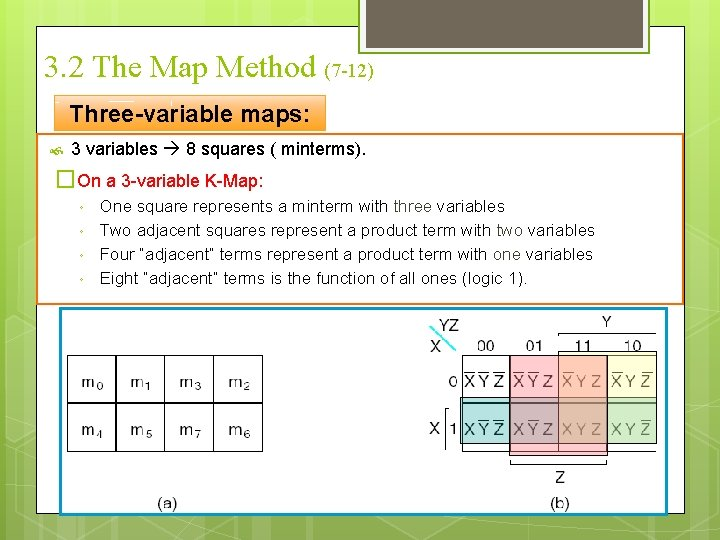3. 2 The Map Method (7 -12) Three-variable maps: 3 variables 8 squares ( minterms). �On a 3 -variable K-Map: ◦ ◦ One square represents a minterm with three variables Two adjacent squares represent a product term with two variables Four “adjacent” terms represent a product term with one variables Eight “adjacent” terms is the function of all ones (logic 1).3. 2 The Map Method (8 -12) Three-variable maps (cont. ): Example 1: F(X, Y) = X’Y’Z’ + X’YZ’ + XY’Z’ + XYZ’ using algebraic manipulations: F = X’Y’Z’ + X’YZ’ + XY’Z’ + XYZ’ = Z’ (X’Y’ + X’Y + XY’ + XY) = Z’ (X’ (Y’+Y) + X (Y’+Y)) = Z’ (X’+ X) = Z’ YZ x 1 13. 2 The Map Method (9 -12) three-Variable maps (cont. ) Example 2: F=AB’C’ +AB C +ABC + A’B’C + A’BC’ � From the map, we see that F=A+B C +BC BC 00 A 0 1 01 11 1 10 1 1 13. 2 The Map Method (10 -12) three-Variable maps (cont. ) Example 4 : F (x, y, z)= Σm (2, 3, 6, 7) � � � using algebraic manipulations: F(x , y, z) = x’yz + x’yz’ + xyz’ = yz (x’ + x) + yz’ (x’ + x) = yz + yz’ = y (z + z’) =y Y yz 11 10 0 1 1 1 00 x 01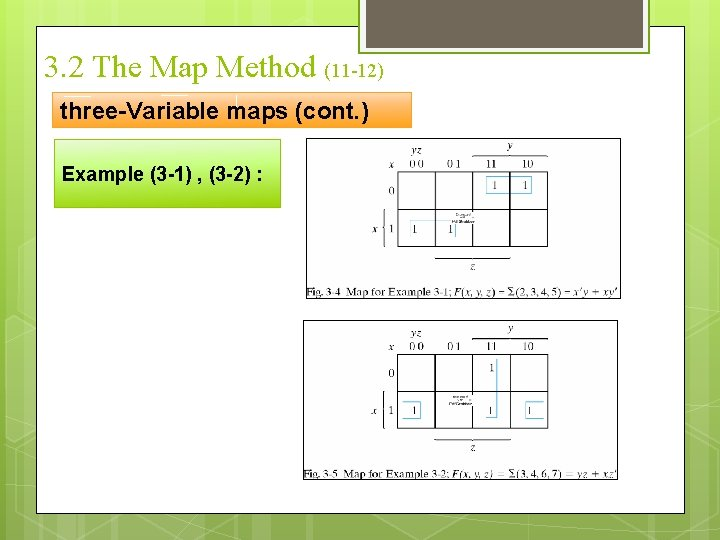3. 2 The Map Method (11 -12) three-Variable maps (cont. ) Example (3 -1) , (3 -2) :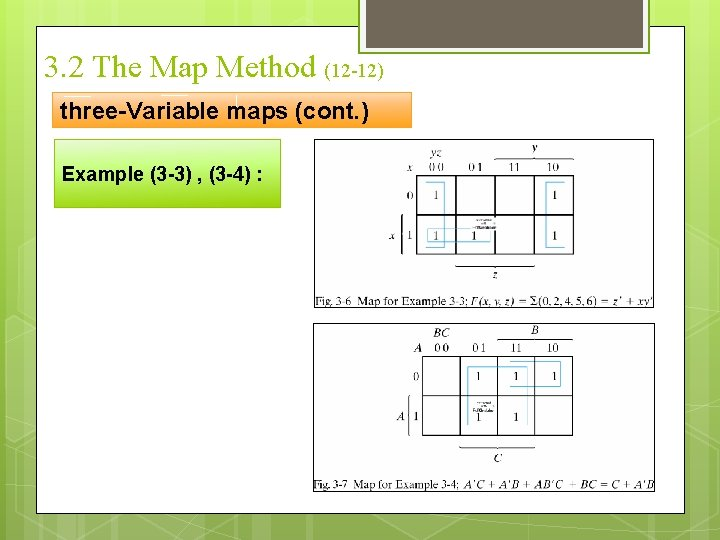3. 2 The Map Method (12 -12) three-Variable maps (cont. ) Example (3 -3) , (3 -4) :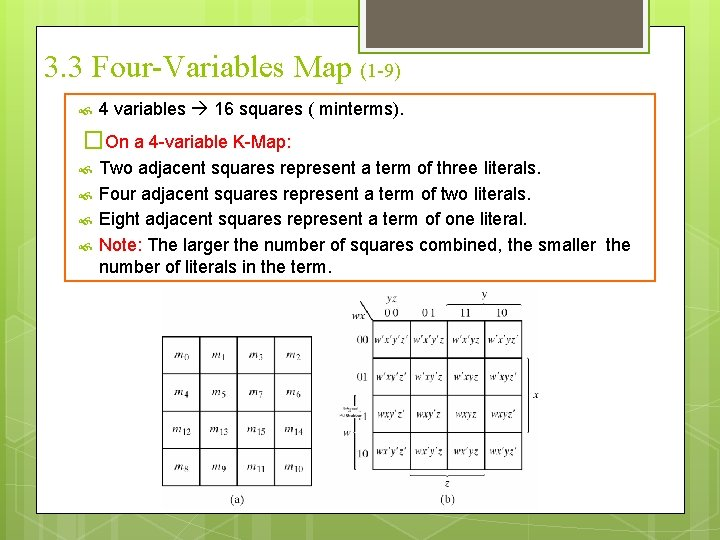3. 3 Four-Variables Map (1 -9) 4 variables 16 squares ( minterms). �On a 4 -variable K-Map: Two adjacent squares represent a term of three literals. Four adjacent squares represent a term of two literals. Eight adjacent squares represent a term of one literal. Note: The larger the number of squares combined, the smaller the number of literals in the term.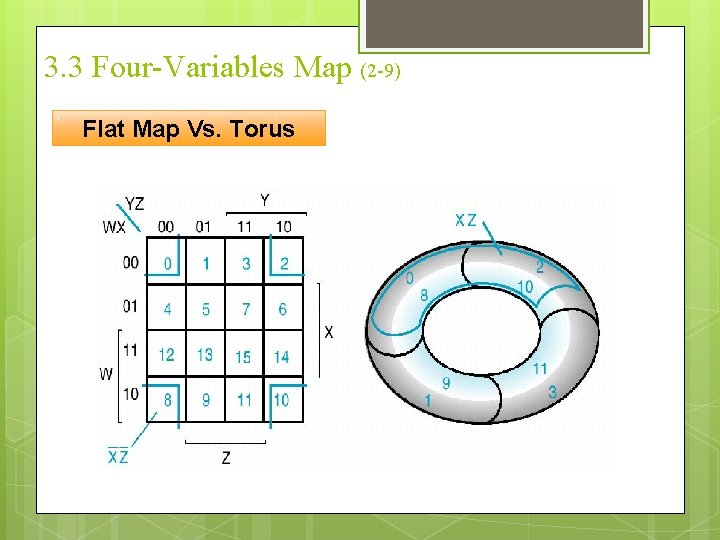3. 3 Four-Variables Map (2 -9) Flat Map Vs. Torus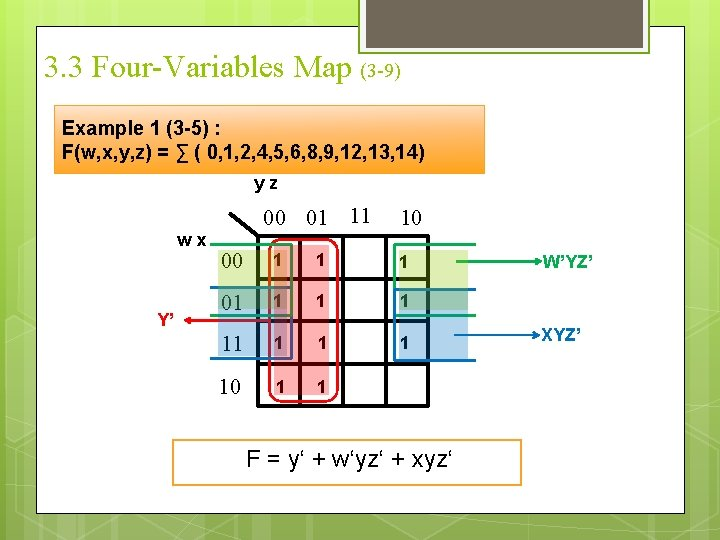3. 3 Four-Variables Map (3 -9) Example 1 (3 -5) : F(w, x, y, z) = ∑ ( 0, 1, 2, 4, 5, 6, 8, 9, 12, 13, 14) yz wx Y’ 00 01 11 10 00 1 1 1 01 1 1 1 10 1 1 F = y‘ + w‘yz‘ + xyz‘ W’YZ’ XYZ’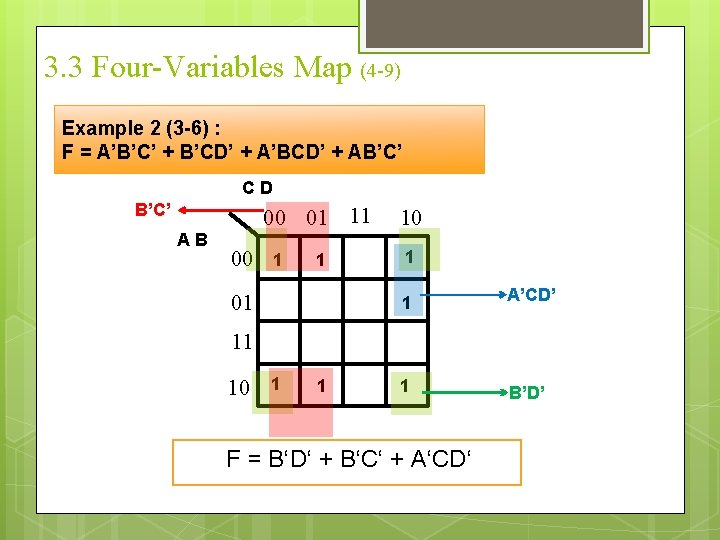3. 3 Four-Variables Map (4 -9) Example 2 (3 -6) : F = A’B’C’ + B’CD’ + A’BCD’ + AB’C’ CD B’C’ AB 00 01 11 00 1 1 01 10 1 1 A’CD’ 1 B’D’ 11 10 1 1 F = B‘D‘ + B‘C‘ + A‘CD‘3. 3 Four-Variables Map (5 -9) Simplification using Prime Implicants o A Prime Implicant is a product term obtained by combining the maximum possible number of adjacent squares in the map. o If a minterm in a square is covered by only one prime implicant, that implicant is called Essential. o Prime Implicants and Essential Prime Implicants can be determined by inspection of a K-Map. o Notes: • Two adjacent 1’s form prime implicant, if they are not within a group of four adjacent squares. • Four adjacent 1’s form prime implicant, if they are not within a group of eight adjacent squares and so on.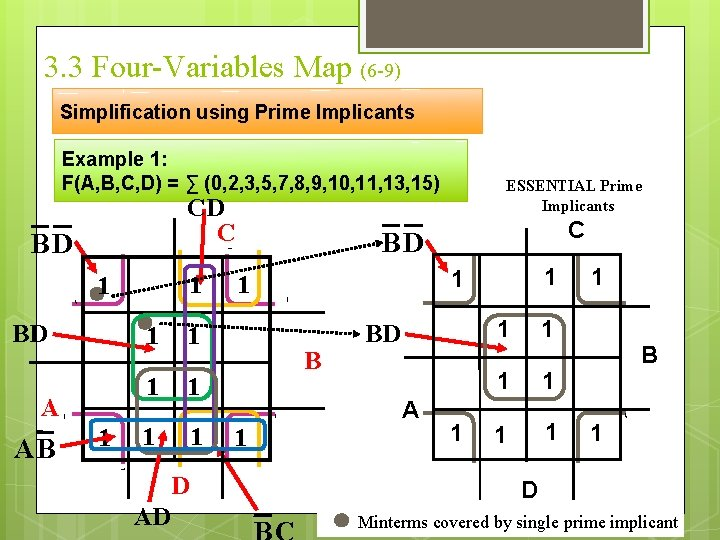3. 3 Four-Variables Map (6 -9) Simplification using Prime Implicants Example 1: F(A, B, C, D) = ∑ (0, 2, 3, 5, 7, 8, 9, 10, 11, 13, 15) CD C BD 1 1 BD A AB 1 1 1 1 D AD ESSENTIAL Prime Implicants C BD B 1 1 1 BD 1 1 B A 1 1 1 D Minterms covered by single prime implicant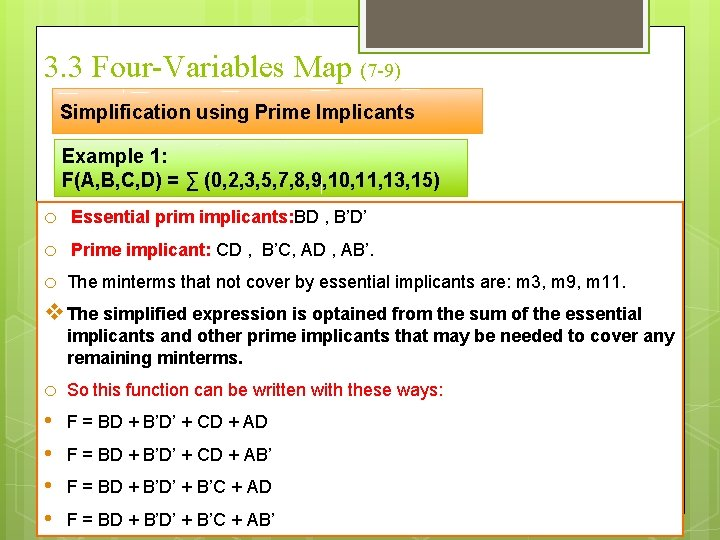3. 3 Four-Variables Map (7 -9) Simplification using Prime Implicants Example 1: F(A, B, C, D) = ∑ (0, 2, 3, 5, 7, 8, 9, 10, 11, 13, 15) o Essential prim implicants: BD , B’D’ o Prime implicant: CD , B’C, AD , AB’. o The minterms that not cover by essential implicants are: m 3, m 9, m 11. v The simplified expression is optained from the sum of the essential implicants and other prime implicants that may be needed to cover any remaining minterms. o • • So this function can be written with these ways: F = BD + B’D’ + CD + AD F = BD + B’D’ + CD + AB’ F = BD + B’D’ + B’C + AD F = BD + B’D’ + B’C + AB’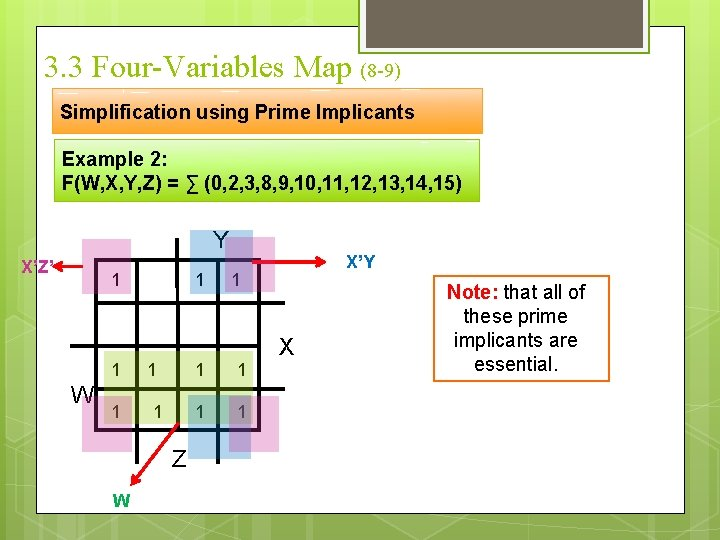3. 3 Four-Variables Map (8 -9) Simplification using Prime Implicants Example 2: F(W, X, Y, Z) = ∑ (0, 2, 3, 8, 9, 10, 11, 12, 13, 14, 15) Y X’Z’ 1 1 W 1 1 Z W X’Y 1 1 1 X Note: that all of these prime implicants are essential.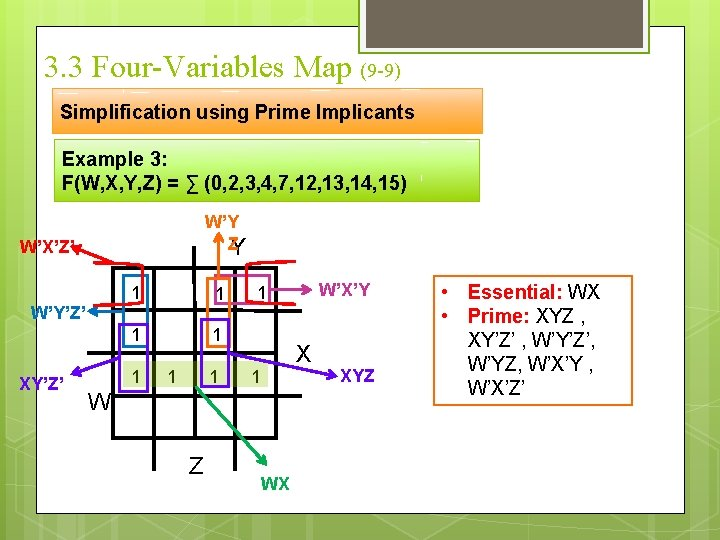3. 3 Four-Variables Map (9 -9) Simplification using Prime Implicants Example 3: F(W, X, Y, Z) = ∑ (0, 2, 3, 4, 7, 12, 13, 14, 15) W’Y Z Y W’X’Z’ W’Y’Z’ XY’Z’ 1 1 1 1 X 1 W Z W’X’Y 1 WX XYZ • Essential: WX • Prime: XYZ , XY’Z’ , W’Y’Z’, W’YZ, W’X’Y , W’X’Z’3. 5 Producut-of-Sum simplification (1 -9) 1. Mark with 1’s the minterms of F. 2. Mark with 0’s the minterms of F’. 3. Circle 0’s to express F’. 4. Complement the result in step 3 to obtain a simplified F in product-of-sums form.3. 5 Producut-of-Sum simplification (1 -9) Example 1: Simplify : F= ∑(0, 1, 2, 5, 8, 9, 10) in Product-of-Sums Form • F’ = AB + CD + BD’ • F = (F’)’ = (A’+B’) + (C’+D’) + (B’+D) CD C BD’ A 1 1 0 1 0 0 0 1 1 0 1 D B AB3. 5 Producut-of-Sum simplification (1 -9) Example 2: Simplify : F(x, y, z) = (0, 2, 5, 7)in Product-of-Sums Form X’Z’ yz 00 x 0 1 11 01 0 10 0 XZ • F’ = XZ + X’Z’ • F = (F’)’ = (X’+Z’) + (X+Z)3. 6 Don't Cares Condition (1 -4) Sometimes a function table or map contains entries for which it is known: § The input values for the minterm will never occur, or § The output value for the minterm is not used. § Functions that have unspecified outputs for some input combinations are called incompletely specified functions. In these cases, the output value is defined as a “don't care” ( an “x” entry) assumed to be either 0 or 1. The choice between 0 and 1 is depending on the way the incompletely specified function is simplied. By placing “don't cares” in the function table or map, the cost of the logic circuit may be lowered.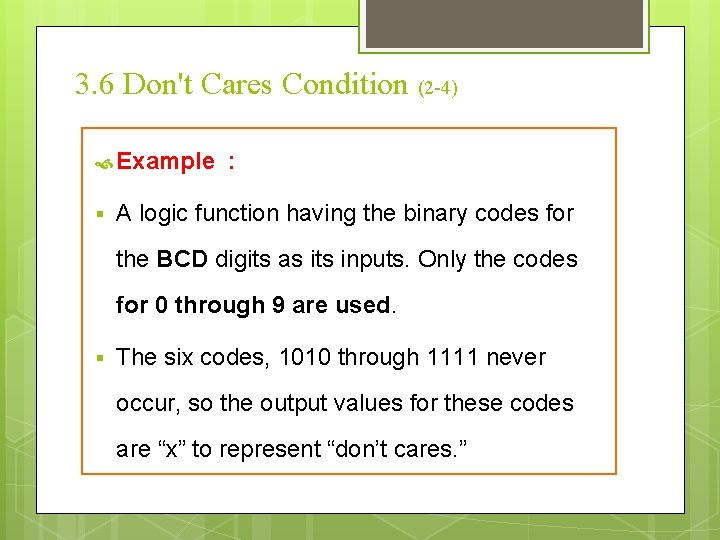3. 6 Don't Cares Condition (2 -4) Example § : A logic function having the binary codes for the BCD digits as its inputs. Only the codes for 0 through 9 are used. § The six codes, 1010 through 1111 never occur, so the output values for these codes are “x” to represent “don’t cares. ”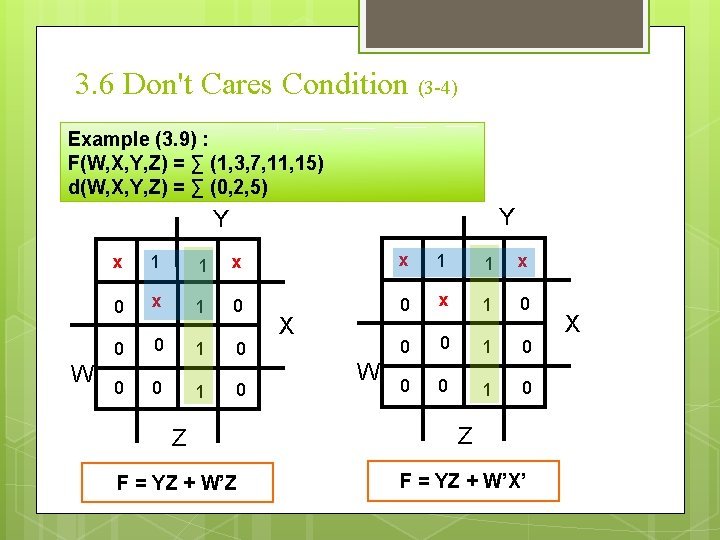3. 6 Don't Cares Condition (3 -4) Example (3. 9) : F(W, X, Y, Z) = ∑ (1, 3, 7, 11, 15) d(W, X, Y, Z) = ∑ (0, 2, 5) Y Y W x 1 1 x 0 x 1 0 0 0 1 0 X W Z Z F = YZ + W’X’ X3. 6 Don't Cares Condition (4 -4) Example (3. 9) : F(W, X, Y, Z) = ∑ (1, 3, 7, 11, 15) d(W, X, Y, Z) = ∑ (0, 2, 5) Y W x 1 1 x 0 x 1 0 0 0 1 0 Z F’ = Z’ + WY’ F = Z ( W’ + Y) X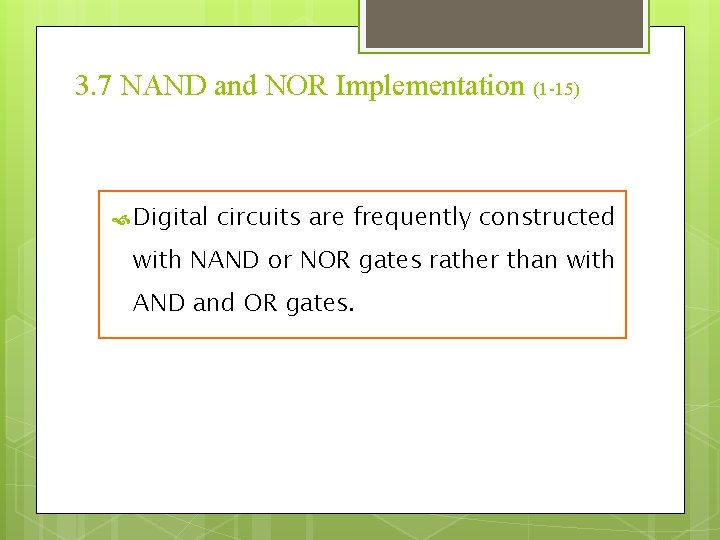3. 7 NAND and NOR Implementation (1 -15) Digital circuits are frequently constructed with NAND or NOR gates rather than with AND and OR gates.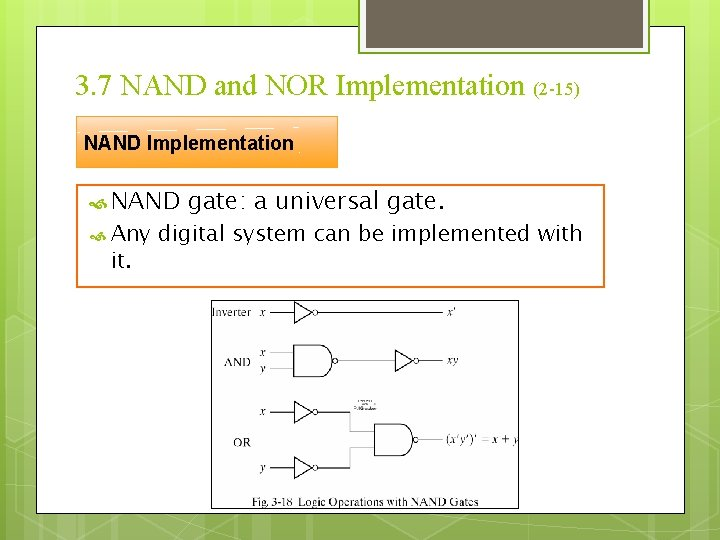3. 7 NAND and NOR Implementation (2 -15) NAND Implementation NAND Any it. gate: a universal gate. digital system can be implemented with3. 7 NAND and NOR Implementation (3 -15) NAND Implementation To facilitate the conversion to NAND logic, there alternative graphic symbol for it.3. 7 NAND and NOR Implementation (4 -15) NAND Implementation Two-Level Implementation 1. 2. 3. 4. Procedures of Implementation with two levels of NAND gates: Express simplified function in sum of products form. Draw a NAND gate for each product term that has at least two literals to constitute a group of first-level gates Draw a single gate using AND-invert or invert-OR in the second level A term with a single literal requires an inverter in the first level.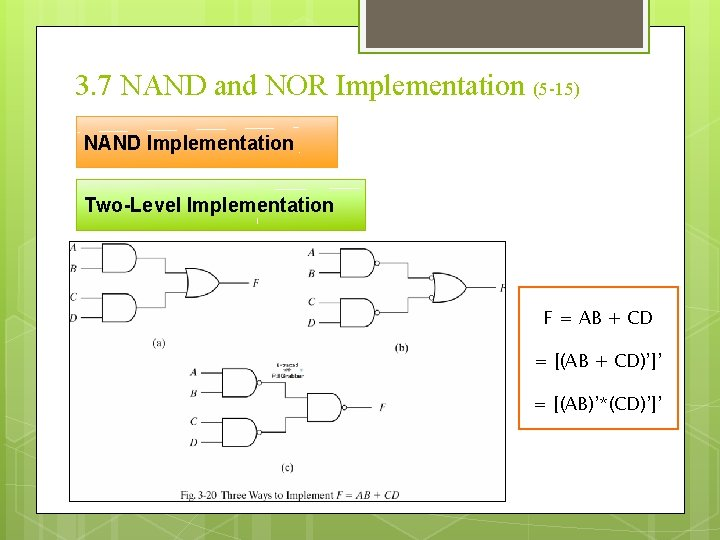3. 7 NAND and NOR Implementation (5 -15) NAND Implementation Two-Level Implementation F = AB + CD = [(AB + CD)’]’ = [(AB)’*(CD)’]’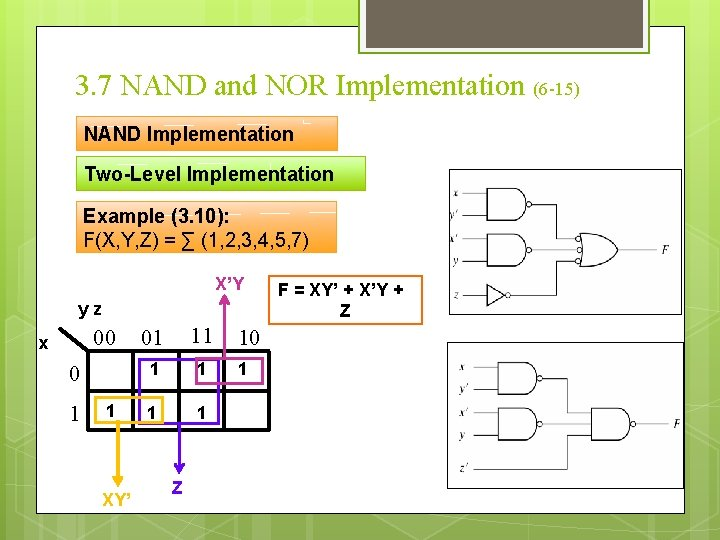3. 7 NAND and NOR Implementation (6 -15) NAND Implementation Two-Level Implementation Example (3. 10): F(X, Y, Z) = ∑ (1, 2, 3, 4, 5, 7) X’Y yz 00 x 0 1 1 XY’ 01 11 1 1 Z 10 1 F = XY’ + X’Y + Z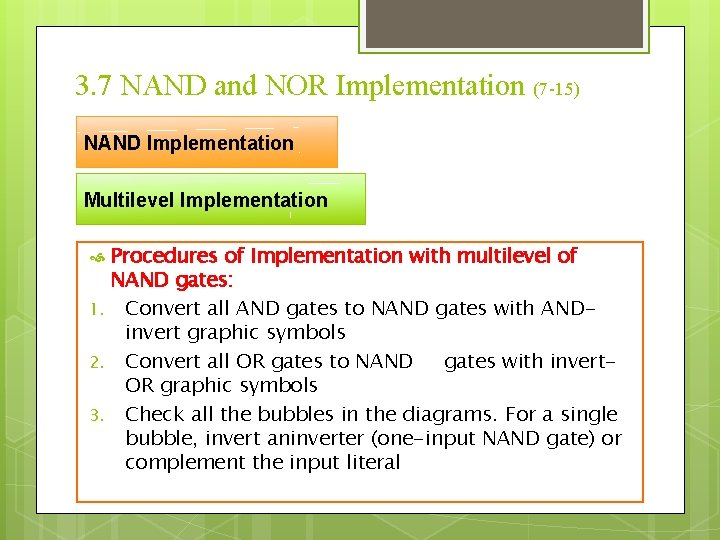3. 7 NAND and NOR Implementation (7 -15) NAND Implementation Multilevel Implementation 1. 2. 3. Procedures of Implementation with multilevel of NAND gates: Convert all AND gates to NAND gates with ANDinvert graphic symbols Convert all OR gates to NAND gates with invert. OR graphic symbols Check all the bubbles in the diagrams. For a single bubble, invert aninverter (one-input NAND gate) or complement the input literal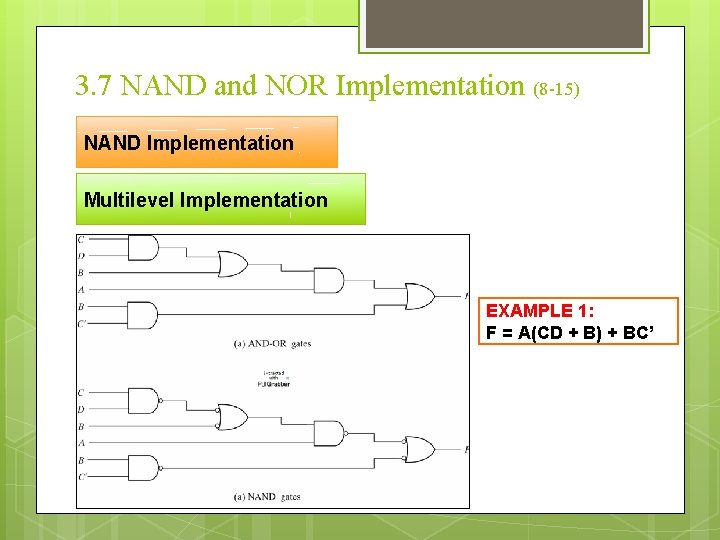3. 7 NAND and NOR Implementation (8 -15) NAND Implementation Multilevel Implementation EXAMPLE 1: F = A(CD + B) + BC’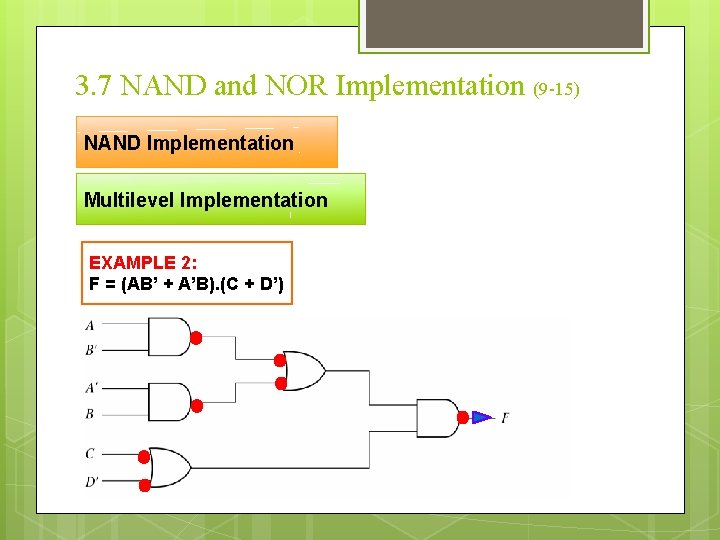3. 7 NAND and NOR Implementation (9 -15) NAND Implementation Multilevel Implementation EXAMPLE 2: F = (AB’ + A’B). (C + D’)3. 7 NAND and NOR Implementation (10 -15) NOR Implementation The NOR operation is the dual of the NAND operation. The NOR gate is anothar universal gate to implement any Boolean function.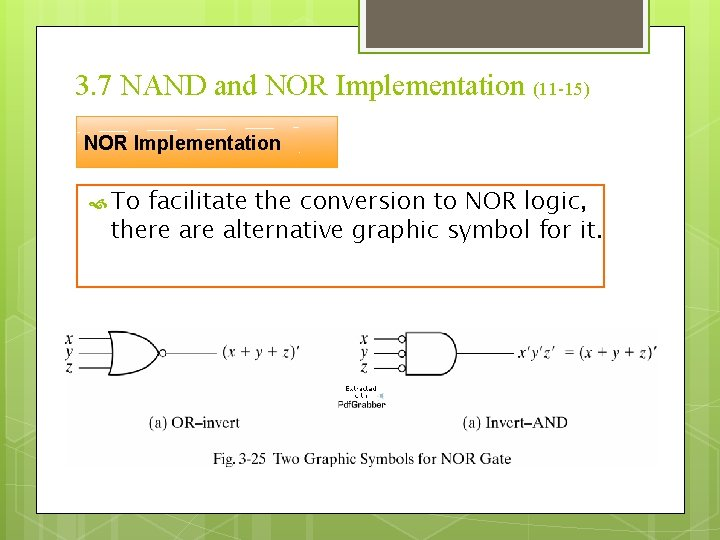3. 7 NAND and NOR Implementation (11 -15) NOR Implementation To facilitate the conversion to NOR logic, there alternative graphic symbol for it.3. 7 NAND and NOR Implementation (12 -15) NOR Implementation Two-Level Implementation 1. 2. 3. 4. Procedures of Implementation with two levels of NOR gates: Express simplified function in product of sums form. Draw a NOR gate for each product term that has at least two literals to constitute a group of first-level gates Draw a single gate using OR-invert or invert-AND in the second level A term with a single literal requires an inverter in the first level.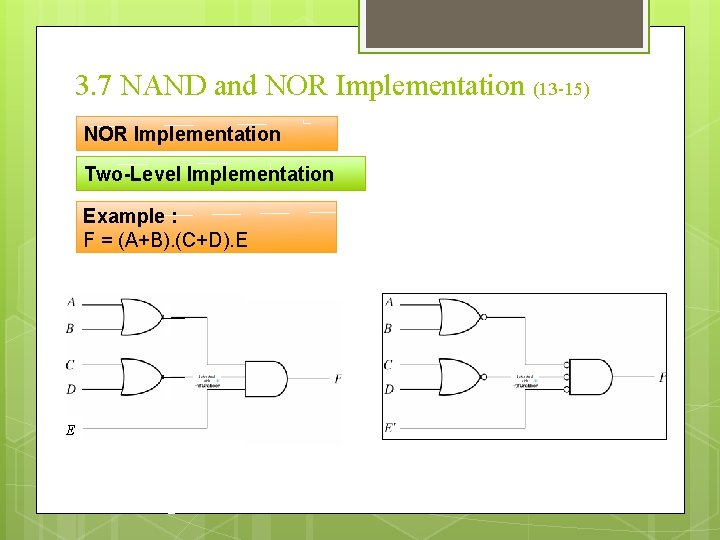3. 7 NAND and NOR Implementation (13 -15) NOR Implementation Two-Level Implementation Example : F = (A+B). (C+D). E E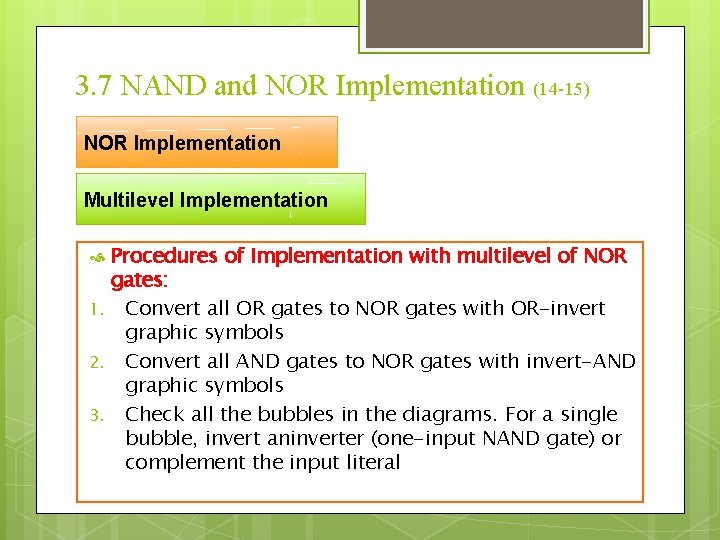3. 7 NAND and NOR Implementation (14 -15) NOR Implementation Multilevel Implementation 1. 2. 3. Procedures of Implementation with multilevel of NOR gates: Convert all OR gates to NOR gates with OR-invert graphic symbols Convert all AND gates to NOR gates with invert-AND graphic symbols Check all the bubbles in the diagrams. For a single bubble, invert aninverter (one-input NAND gate) or complement the input literal3. 7 NAND and NOR Implementation (15 -15) NOR Implementation Multi-Level Implementation Example : F = (A B’ + A’B). (C+D’) A B’ A’ B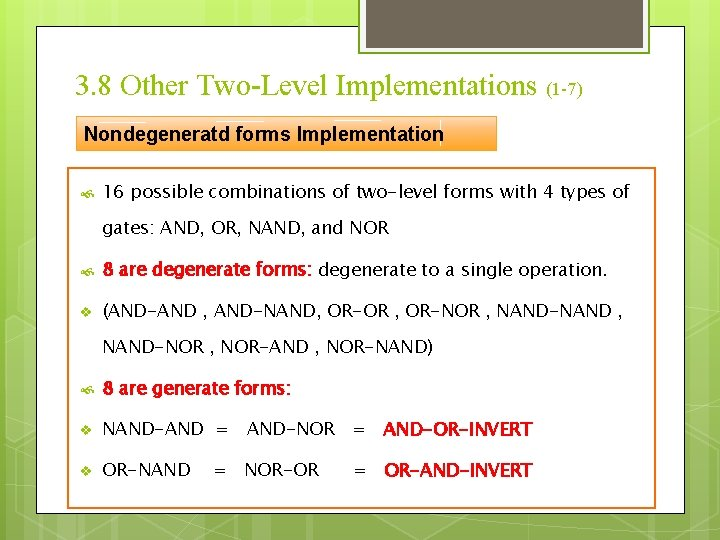3. 8 Other Two-Level Implementations (1 -7) Nondegeneratd forms Implementation 16 possible combinations of two-level forms with 4 types of gates: AND, OR, NAND, and NOR 8 are degenerate forms: degenerate to a single operation. v (AND-AND , AND-NAND, OR-OR , OR-NOR , NAND-NAND , NAND-NOR , NOR-AND , NOR-NAND) 8 are generate forms: v NAND-AND = AND-NOR = AND-OR-INVERT v OR-NAND = NOR-OR = OR-AND-INVERT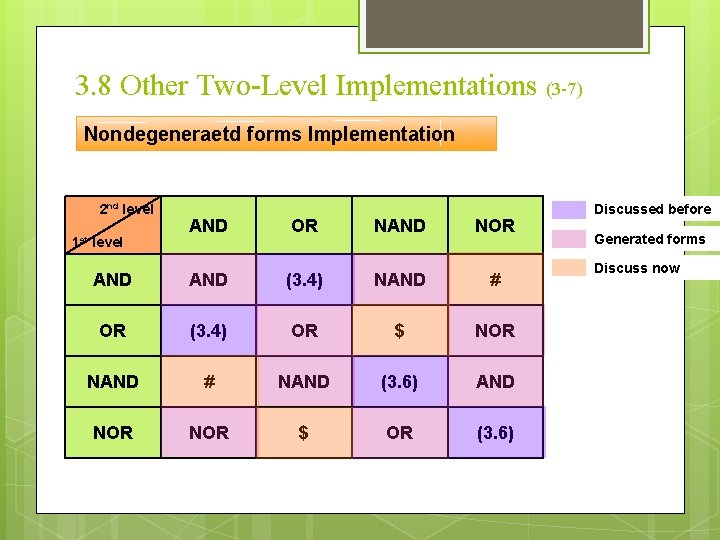3. 8 Other Two-Level Implementations (3 -7) Nondegeneraetd forms Implementation 2 nd level AND OR NAND NOR AND (3. 4) NAND # OR (3. 4) OR \$ NOR NAND # NAND (3. 6) AND NOR \$ OR (3. 6) 1 st level Discussed before Generated forms Discuss now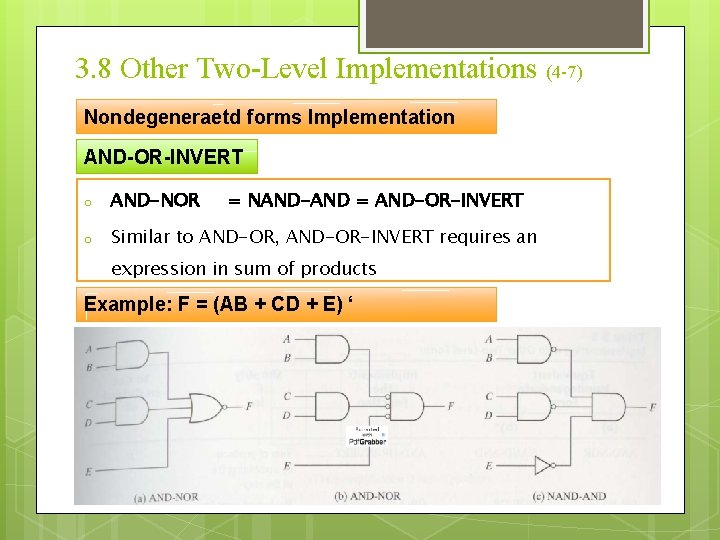3. 8 Other Two-Level Implementations (4 -7) Nondegeneraetd forms Implementation AND-OR-INVERT o AND-NOR = NAND-AND = AND-OR-INVERT o Similar to AND-OR, AND-OR-INVERT requires an expression in sum of products Example: F = (AB + CD + E) ‘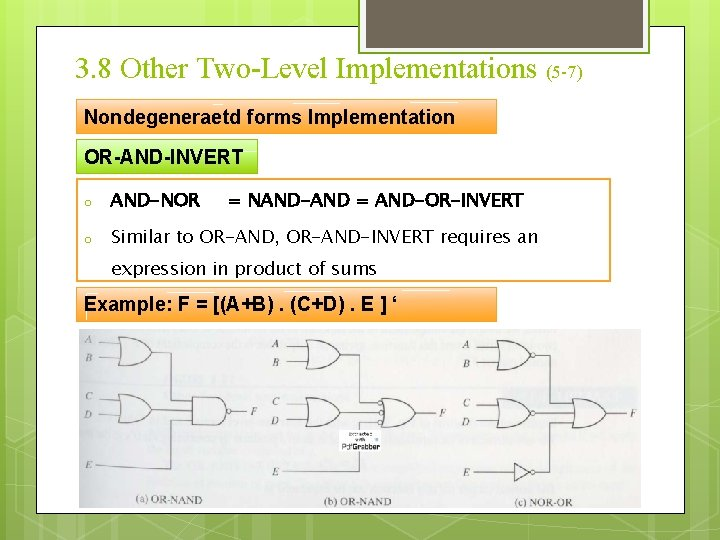3. 8 Other Two-Level Implementations (5 -7) Nondegeneraetd forms Implementation OR-AND-INVERT o AND-NOR = NAND-AND = AND-OR-INVERT o Similar to OR-AND, OR-AND-INVERT requires an expression in product of sums Example: F = [(A+B). (C+D). E ] ‘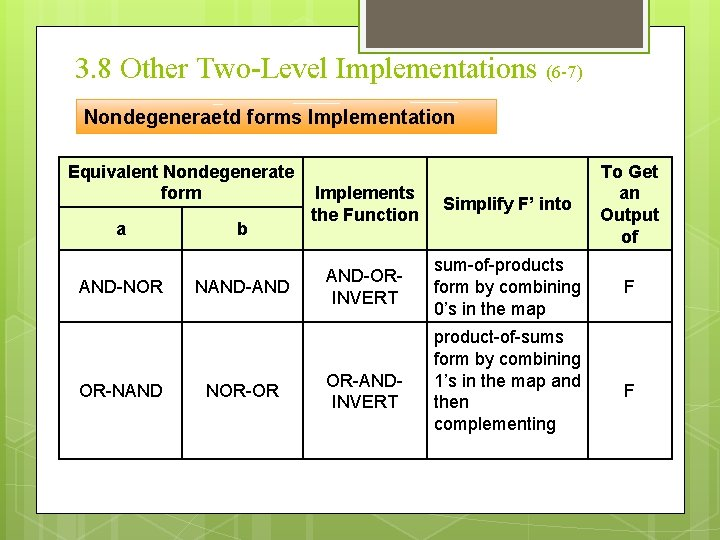3. 8 Other Two-Level Implementations (6 -7) Nondegeneraetd forms Implementation Equivalent Nondegenerate form a AND-NOR OR-NAND b NAND-AND NOR-OR Implements the Function Simplify F’ into To Get an Output of AND-ORINVERT sum-of-products form by combining 0’s in the map F OR-ANDINVERT product-of-sums form by combining 1’s in the map and then complementing F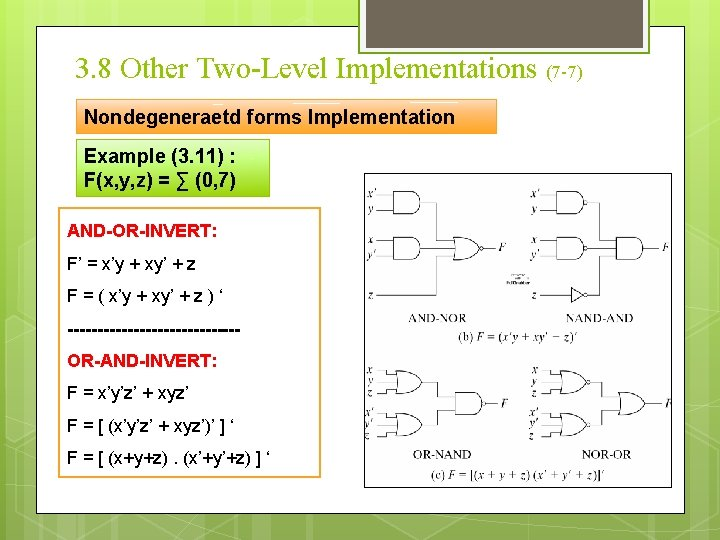3. 8 Other Two-Level Implementations (7 -7) Nondegeneraetd forms Implementation Example (3. 11) : F(x, y, z) = ∑ (0, 7) AND-OR-INVERT: F’ = x’y + xy’ + z F = ( x’y + xy’ + z ) ‘ --------------OR-AND-INVERT: F = x’y’z’ + xyz’ F = [ (x’y’z’ + xyz’)’ ] ‘ F = [ (x+y+z). (x’+y’+z) ] ‘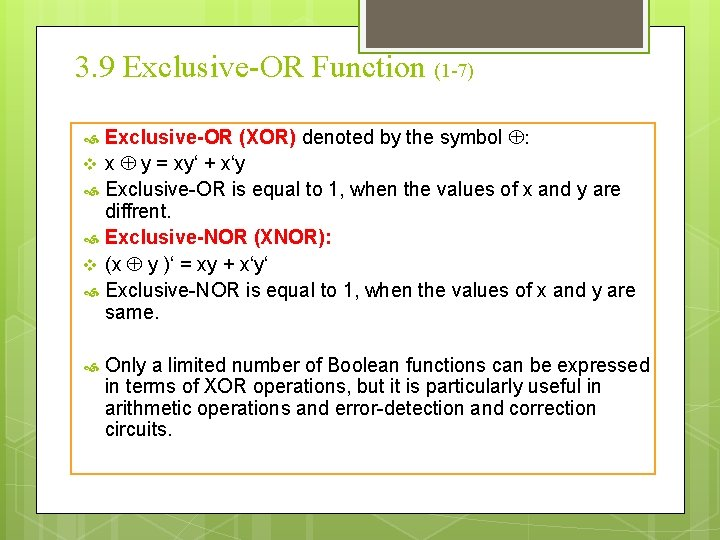3. 9 Exclusive-OR Function (1 -7) Exclusive-OR (XOR) denoted by the symbol : v x y = xy‘ + x‘y Exclusive-OR is equal to 1, when the values of x and y are diffrent. Exclusive-NOR (XNOR): v (x y )‘ = xy + x‘y‘ Exclusive-NOR is equal to 1, when the values of x and y are same. Only a limited number of Boolean functions can be expressed in terms of XOR operations, but it is particularly useful in arithmetic operations and error-detection and correction circuits.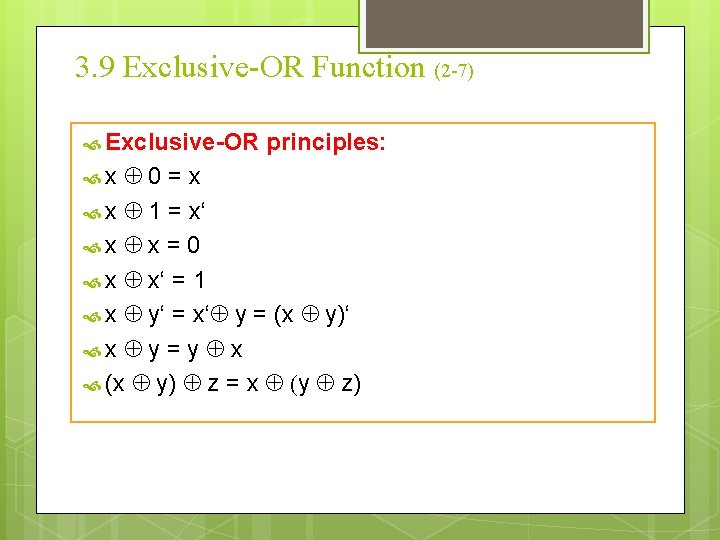3. 9 Exclusive-OR Function (2 -7) Exclusive-OR principles: 0=x x 1 = x‘ x x = 0 x x‘ = 1 x y‘ = x‘ y = (x y)‘ x y = y x (x y) z = x (y z) x3. 9 Exclusive-OR Function (3 -7) Implementaion Exclusive-OR with AND-OR-NOT: x y = xy‘ + x‘y Implementaion Exclusive. OR with NAND: x y = xy‘ + x‘y = x (x‘+y‘) + y (x‘+y‘) = x (xy)‘ + y (xy)‘ = [ (x(xy)‘ + y(xy)‘)‘]‘ = [ (x(xy)‘)‘ + (y(xy)‘)‘ ]‘3. 9 Exclusive-OR Function (4 -7) Odd Function: The 3 -variable XOR function is equal to 1 if only one variable is equal to 1 or if all three variables are equal to 1. Multiple-variable exclusive OR operation = odd function : odd number of variables be equal to 1. (A B C) = (AB‘ + A‘B) C‘ + (A‘B‘ + AB) C = AB‘C‘ + A‘B‘C + ABC = ∑ (1, 2, 4, 7)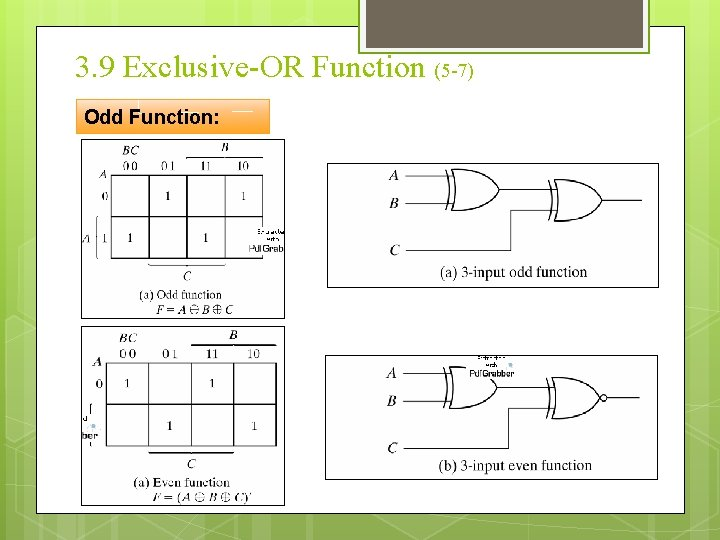3. 9 Exclusive-OR Function (5 -7) Odd Function:3. 9 Exclusive-OR Function (6 -7) Odd Function: A B C D= ∑ (1, 2, 4, 7, 8, 11, 13, 14)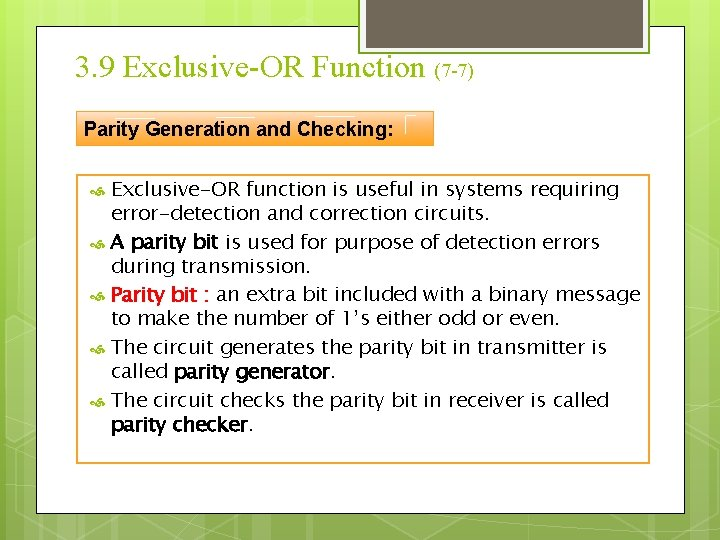3. 9 Exclusive-OR Function (7 -7) Parity Generation and Checking: Exclusive-OR function is useful in systems requiring error-detection and correction circuits. A parity bit is used for purpose of detection errors during transmission. Parity bit : an extra bit included with a binary message to make the number of 1’s either odd or even. The circuit generates the parity bit in transmitter is called parity generator. The circuit checks the parity bit in receiver is called parity checker.3. 9 Exclusive-OR Function (8 -7) Parity Generation and Checking: Example : Three-bit message with even parity Three-bit Massage Parity bit X Y Z P 0 0 0 1 1 0 1 0 1 0 0 1 1 0 0 1 1 o From the truth table , P constitutes an odd function. o It is equal 1 when numerical value of 1’s in a minterm is odd o P=x y z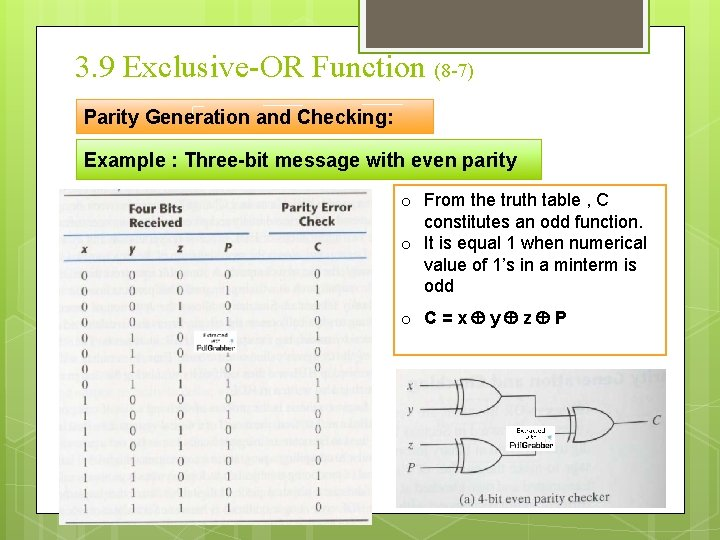3. 9 Exclusive-OR Function (8 -7) Parity Generation and Checking: Example : Three-bit message with even parity o From the truth table , C constitutes an odd function. o It is equal 1 when numerical value of 1’s in a minterm is odd o C=x y z P